# SRMJEEE Maths Mock Test - 5

## 35 Questions MCQ Test SRMJEEE Subject Wise & Full Length Mock Tests | SRMJEEE Maths Mock Test - 5

Description
Attempt SRMJEEE Maths Mock Test - 5 | 35 questions in 50 minutes | Mock test for JEE preparation | Free important questions MCQ to study SRMJEEE Subject Wise & Full Length Mock Tests for JEE Exam | Download free PDF with solutions
QUESTION: 1

Solution:
QUESTION: 2

Solution:
QUESTION: 3

### Let f(x) = x - |x - x2|, x ∈[-1, 1]. Then the number of points at which f(x) is discontinuous is

Solution:

f(x)= x - |x|. |1-x|. We know that x, |x|, |1-x| are continuous everywhere. As the product and algebraic sum of continuous functions are continuous, f(x) is continuous everywhere.

QUESTION: 4

If two circles of equal radii a and with centres (2,3) and (5,6) cut each other at right angle then, a=

Solution:
QUESTION: 5

If in the expansion of [(x4)+(1/x3)]15 in rth term x4 occurs, then r=

Solution:
QUESTION: 6

The correct statement regarding the position of point (-6,2) with respect to lines 2x+3y-4=0 and 6x+9y+8=0 is

Solution:
QUESTION: 7

Equation [(x2)/(2-r)]+[(y2)/(r-5)]+1=0 represents an ellipse if

Solution:
QUESTION: 8

[(1-2i)/(2+i)] + [(4-i)/(3+2i)] =

Solution:
QUESTION: 9

The area bounded by two curves y2=4ax and x2=4ay is

Solution:

Given, y2 = 4ax -- (1)
x2 = 4ay ---(2)
(1) and (2) intersects

hence
x = y2/4a (a > 0)

(y2/4a)2 = 4ay
y4 = 64a3y
y4 – 64a3y = 0
y[y3 – (4a)3] = 0
y = 0, 4a
When y = 0, x = 0 and                     when y = 4a, x = 4a.
The points of intersection of (1) and (2) are O(0, 0) and A(4a, 4a).
The area of the region between the two curves
= Area of the shaded region
04a(y1 – y2)dx
04a[√(4ax) – x2/4a]dx
= [2√a.(x3/2)/(3/2) – (1/4a)(x3/3)] -04a
= 4/3√a(4a)3/2 – (1/12a)(4a)3 – 0
= 32/3a2 – 16/3a2
= 16/3a2 sq. units

QUESTION: 10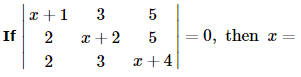Solution:
QUESTION: 11

The degree and order of the differential equation of the family of all parabolas whose axis is x-axis are respectively

Solution:
QUESTION: 12

f(x)=x2-27x+5 is increasing when

Solution:
QUESTION: 13

∫[(1)/(1-sinx)]dx=

Solution:
QUESTION: 14

If cot⁻1[(cos α)1∕2] - tan⁻1[(cot α)1∕2] = x, then sin x =

Solution:
QUESTION: 15

All diagonal elements of skew symmetric matrix are

Solution:
QUESTION: 16

The value of lim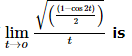Solution:
QUESTION: 17

Let f(x) be a function such that f'(a) ≠ 0. Then at x = a,f(x)

Solution:

Let f(x) = |x|.
f'(0) ≠ 0 but f(x) has a minimum at x = 0.

QUESTION: 18

The inverse of matrix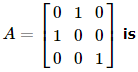Solution:
QUESTION: 19

The number of partition values in case of quartiles is:

Solution:
QUESTION: 20

Given the following data:
No. of observation = 1000
Arithmetic mean = 1000
Variance = 256.0
The co-efficient of variance will be equal to

Solution:
QUESTION: 21

The equation of pair of lines passes through (1,1) and perpendicular to lines 3x2-7xy-2y2=0 is

Solution:
QUESTION: 22

In an election there are five candidates for three places. A man can vote for maximum number of candidates. In how many total ways can a men vote?

Solution:
QUESTION: 23

The probability that a card drawn from a pack of 52 cards will be diamond or king is

Solution:
QUESTION: 24

In a Δ ABC , the inradius and three exradii are r , r1 , r2 and r3 respectively. In usual notations the value of r. r1.r2.r3 is equal to

Solution: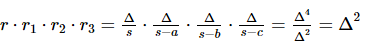QUESTION: 25

If α, β are the roots of the equation x2 + x + 1 = 0 and (α/β), (β/α) are the roots of x2 + px + q = 0, then p is equal to

Solution:
QUESTION: 26

The sum of the series 1.32+ 2.52 + 3.72 +.....upto 20 terms is

Solution:
QUESTION: 27

In the set W of whole numbers an equivalence relation R is defined as follows :

aRb iff both a and b leave same remainder when divided by 5. The equivalence class of 1 is given by

Solution:
QUESTION: 28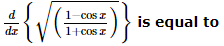Solution:
QUESTION: 29

If lines 2x+3ay-1=0 and 3x+4y+1=0 are metually perpendicular, then a =

Solution:
QUESTION: 30

The tangent to a given curve y=f(x) is perpendicular to x-axis if

Solution:
QUESTION: 31

A random variable X has the following distribution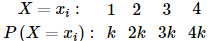The value of k and P X < 3 are equal to

Solution:
QUESTION: 32

If sec4θ-sec2θ=2, the general value of θ is

Solution:
QUESTION: 33

The value of cos2 π/12+cos2π/4+cos25π/12 is

Solution:
QUESTION: 34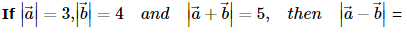Solution:
QUESTION: 35

Vector B=3j+4k, is equal to the sum of B₁ and B₂. If B₁ and B₂ are parallel and perpendicular to A=i+j, then B₁=

Solution:Use Code STAYHOME200 and get INR 200 additional OFF Use Coupon Code